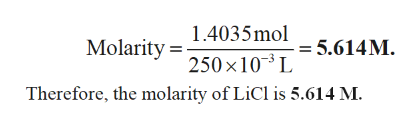# Calculate the molarity of each of the following: 59.5 g LiCl in 250.0 mL of solution and 32.0 g NiSO4 in 200.0 mL of solution.

Question
188 views

Calculate the molarity of each of the following: 59.5 g LiCl in 250.0 mL of solution and 32.0 g NiSO4 in 200.0 mL of solution.

check_circle

Step 1

Molarity of LiCl:

Number of moles of LiCl = mass / molar mass = 59.5 g / 42.394 g mol-1 = 1.40...help_outlineImage Transcriptionclose1.4035mol 5.614M L Molarity 250 x 10 Therefore, the molarity of LiCl is 5.614 M. fullscreen

### Want to see the full answer?

See Solution

#### Want to see this answer and more?

Solutions are written by subject experts who are available 24/7. Questions are typically answered within 1 hour.*

See Solution
*Response times may vary by subject and question.
Tagged in

### Physical Chemistry